Tutorial math and reading software for elementary and secondary arithmetic, basic math, algebra, geometry, precalculus plus GED, ABE, and CLEP preparation for elementary school, high school, college, adult education, and homeschool students.

# Arithmetic SeriesProgram 4: MULTIPLICATIONLearn the Multiplication Math Facts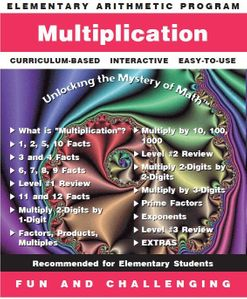Item# MULT-Win
Regular price: \$49.00
Limited Time Offer: \$24.50
Media type::

## Product Description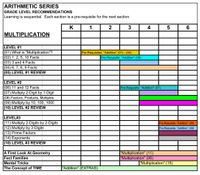[click on images]

Math software to teach math multiplication -- The multiplication facts are learned in a variety of ways - First the student studies the 1, 2, 5, and 10 multiplication facts by counting, then by multiples, then in multiplication tables. Follow up each computer math practice of the math facts with multiplication worksheets. The second group of multiplication facts are the 3 and 4 facts - with multiplication problems that point out the association between addition and multiplication. With each level, the student fills in even larger multiplication tables. The next multiplication lesson is the 6, 7, 8, and 9 multiplication facts. Once the student has practiced all these facts, they are given the opportunity to show their achievement in a variety of multiplication tables. Use the printable multiplication worksheets for class work or homework to reinforce the multiplication lessons and math facts in the software program. Blank multiplication tables are printable from the multiplication worksheets for additional math practice.

This is the 4th software program in the Arithmetic Series. Math facts are practiced within the program and also in multiplication worksheets. It is packed with learning opportunities and math practice in, not only all aspects of multiplying whole numbers, from learning and practicing the multiplication facts through 12, multiplying by 2 and 3 digit numbers, prime factorization and exponents; but also a massive geometry section for beginning geometry students (points, lines, angles, coordinate geometry, perimeter, and area).

## Accessories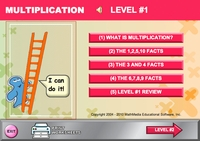Multiplication - Level #1 - MENU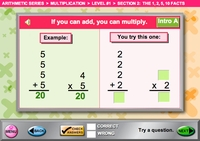Learn the connection between addition and multiplication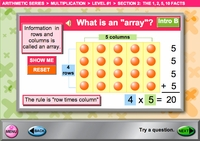Another perspective on multiplication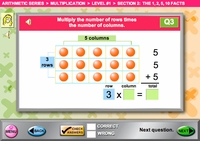Practice each concept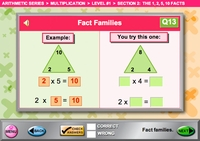Learn factors and product method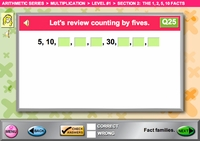Learn the multiples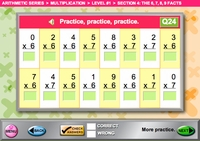Drill the facts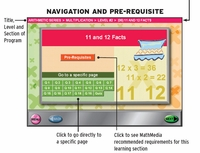Navigation page begins each section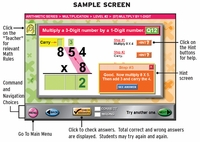Each step unfolds - on demand from the student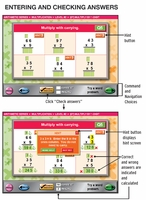Checking answers